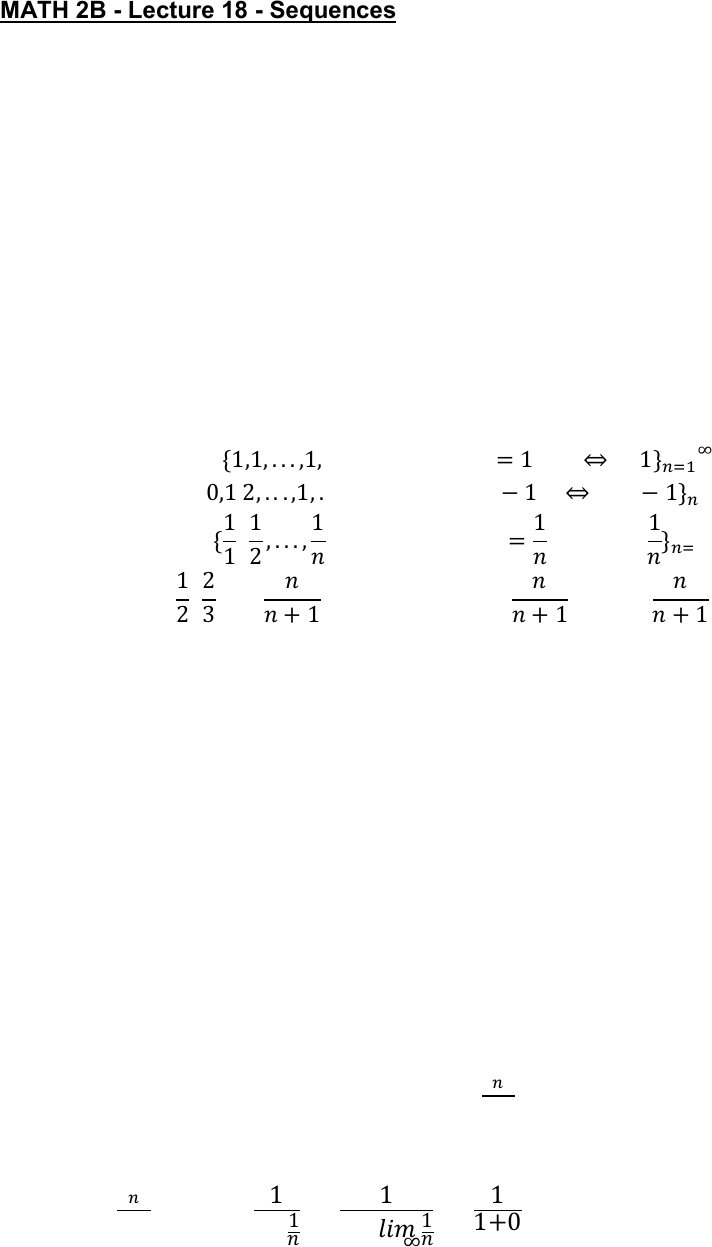Class Notes (1,100,000)
US (460,000)
UC-Irvine (10,000)
MATH (1,000)
MATH 2B (600)
Lecture 18

# MATH 2B Lecture 18: SequencesPremium

Department
Mathematics
Course Code
MATH 2B
Professor
ERJAEE, G.
Lecture
18

This preview shows half of the first page. to view the full 3 pages of the document.02/15/2019
MATH 2B - Lecture 18 - Sequences
Sequence - a list of infinite numbers written in a definite order
Notations - 
or  or 
Example:
 can be denoted as:
 
preceding notation general formula explicit
expression
Example: 










Divergence & Convergence
A sequence  is convergent, if 
, L is a finite number
Otherwise, we say  is divergent
Basically, we try to find the general formula for a sequence, , then try to
find 


If 
 is finite  is convergent. Otherwise,  is divergent
Example:
Determine whether
 is convergent
Solution:







[Convergent]